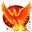#wx.FontWeight¶

The FontWeight enumeration provides the following values:

Description

Value

wx.FONTWEIGHT_INVALID

Invalid font weight.

wx.FONTWEIGHT_THIN

Thin font (weight = 100).

wx.FONTWEIGHT_EXTRALIGHT

Extra Light (Ultra Light) font (weight = 200).

wx.FONTWEIGHT_LIGHT

Light font (weight = 300).

wx.FONTWEIGHT_NORMAL

Normal font (weight = 400).

wx.FONTWEIGHT_MEDIUM

Medium font (weight = 500).

wx.FONTWEIGHT_SEMIBOLD

Semi Bold (Demi Bold) font (weight = 600).

wx.FONTWEIGHT_BOLD

Bold font (weight = 700).

wx.FONTWEIGHT_EXTRABOLD

Extra Bold (Ultra Bold) font (weight = 800).

wx.FONTWEIGHT_HEAVY

Heavy (Black) font (weight = 900).

wx.FONTWEIGHT_EXTRAHEAVY

Extra Heavy font (weight = 1000).

wx.FONTWEIGHT_MAX